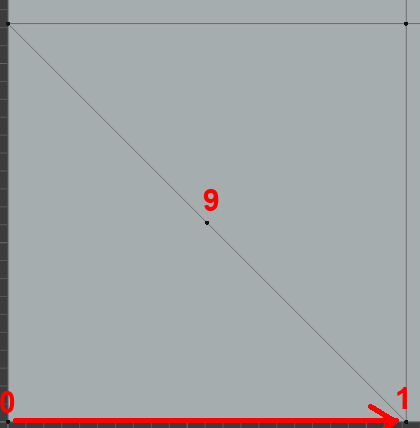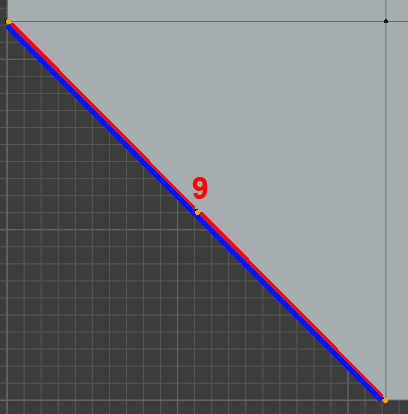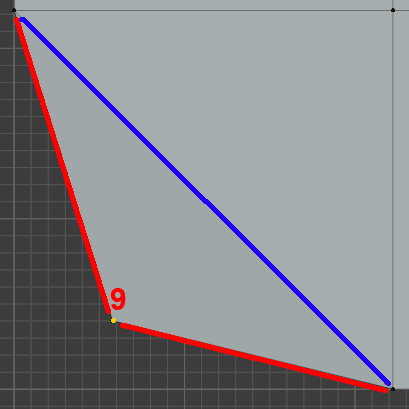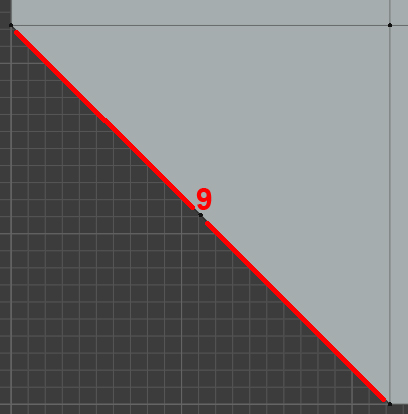# Problems using bmesh.utils.vert_collapse_faces

Hi devs, I’m working on video tutorials for bmesh’s utilities and operators and got stuck on bmesh.utils.vert_collapse_faces. I’m facing few misunderstandings probably.

Issue 1
How is bmesh.utils.vert_collapse_faces different from bmesh.utils.vert_collapse_edge?

If I overlook the fact that vert_collapse_faces has two more parameters for now, what you see below is exactly the same result as with using vert_collapse_edge.

Issue 2
Documentation says “fac (float) – The factor to use when merging customdata [0 - 1]”

To test this parameter I assume that customdata is eg. vertex weights. Using the same example as above, I assigned weight=1 to all vertices except vert, which has weight=0.

My understanding is that if fac is:
0 then weight of vert remains 1, original vert has zero influence on vert
1 then weight of vert changes to 0 as original vert has 100% influence on vert
But I still get the same(original) value 1, whatever fac I set. Is it buggy or I’m getting it wrong?

Issue 3
Parameter join_faces isn’t documented. - patch is here https://developer.blender.org/D9596
vert_collapse_faces works for me only if join_faces is set to False, using True I get
`ValueError: vert_collapse_faces(vert, edge): no new edge created, internal error`
Can you help me understand what it is suppose to do.

I checked github, blenderartists forums, bug tracker, addons bundled with Blender, but I couldn’t find a single example of how to use vert_collapse_faces properly.

Devs, pretty please, give me a hand.

Hi, they are quite similar, the main difference is, as you’ve noticed, internally `vert_collapse_faces` calls `BM_vert_collapse_faces` can be called with `join_faces=true`.

`````` * \param join_faces: When true the faces around the vertex will be joined
* otherwise collapse the vertex by merging the 2 edges this vert touches into one.
``````

(added this to the Python docs)

Thank you for the docstring @ideasman42, I think I’ve found out the edge case when join_faces set to True becomes handy, but instead of being handy it throws an error.

In case when collapsing vertex 0 into vertex 1, having join_faces set to Falsenewly created edge(blue) lines up perfectly with two existing edges(red)the situation is easier to understand if vertex 9 is moved a bit off the edgeNow, is my assumption correct that to get result without the “blue” edge(pic below), I should use join_faces set to True?If I actually run the script with join_faces is set to True, in the case described above I get an error:
`ValueError: vert_collapse_faces(vert, edge): no new edge created, internal error`